×#### Thank you for registering.

One of our academic counsellors will contact you within 1 working day.

Click to Chat

1800-1023-196

+91-120-4616500

CART 0

• 0

MY CART (5)

Use Coupon: CART20 and get 20% off on all online Study Material

ITEM
DETAILS
MRP
DISCOUNT
FINAL PRICE
Total Price: Rs.

There are no items in this cart.
Continue Shopping• Complete JEE Main/Advanced Course and Test Series
• OFFERED PRICE: Rs. 15,900
• View Details

```Revision Notes on Visualising Solid Shapes

Plane Figures (2-Dimensional Shape)

The figures which we can draw on a flat surface are called Plane Figures. They have two dimensions i.e. length and breadth, hence these are called 2 Dimensional Shapes.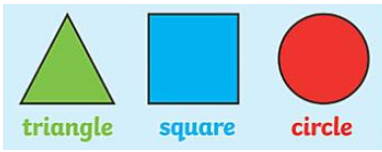Solid Figures (3-Dimensional Shape)

The figures which have three dimensions i.e. length, breadth and height are called 3 –Dimensional Shape. They occupy some space. Like the ball, box, tube etc.Faces, Edges and VerticesFaces – All the flat surfaces of the 3-D figure are the faces of that shape. The faces of 3D shapes are made by the 2-D shapes.

Edges – The line segment where the faces of the 3D shape meet with each other are the edges of that shape.

Vertices – The corners or the points where the edges meet with each other are the vertices of the 3D shape. The singular form of vertices is the vertex.

No. of Faces, Edges and Vertices in some common 3- D Shapes

S.No.
3 – D shape
Figure
Faces
Edges
Vertices

1.
Cube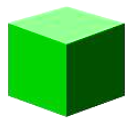6
12
8

2.
Cuboid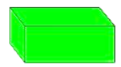6
12
8

3.
Cone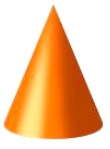2
1
1

4.
Sphere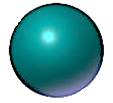1
1
0

5.
Cylinder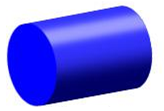3
2
0

6.
Square based Pyramid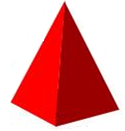6
8
5

Nets for building 3 – D Shapes

If we draw the structure on the 2D form and fold it to make a 3D shape then it is said to be the net of that figure. Different figures have a different type of nets.

We can open a 3D shape from its edges to get the net of that figure.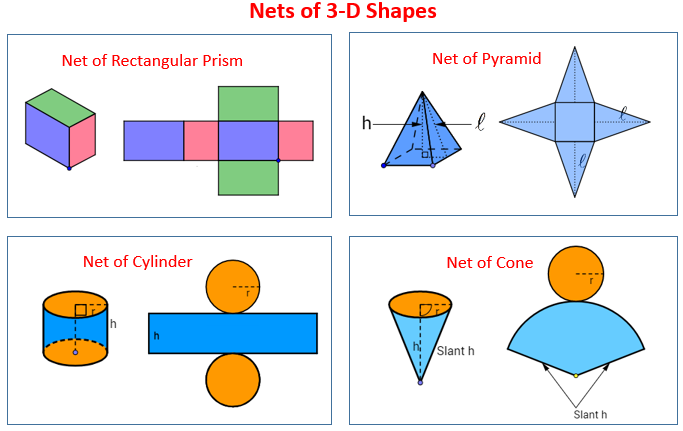Drawing Solids on a Flat Surface (2D representation of 3D Shapes)

As you know that the 3D shapes are the shapes which occupy some space but we can draw the 3D shapes on the flat surface also by some techniques. This is called a visual illusion.

1. Oblique Sketches

When we draw a shape in such a way that we are not able to see some of the faces of the 3D shape and the size of the length is also not equal but we are able to recognize that this is a cube then this is called an Oblique Sketch.

Steps to draw an Oblique Sketch

Draw the sketch of a cube with a side of 4 cm each.

Step 1: First, we need to draw a square of 2 × 2 on a grid sheet.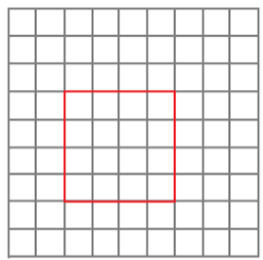Step 2: Draw the opposite face of the same size which is offset to the front face.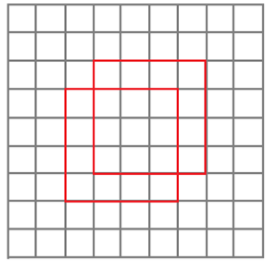Step 3: Now, join the respective corners, to form a cube.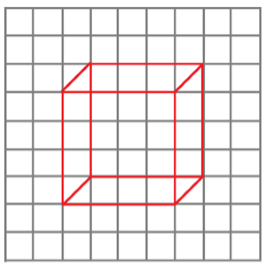Points to remember for the oblique sketch-

The front and back faces are of the same size.

All the edges are appearing as of the same length but their measurements are different.

2. Isometric Sketches

The isometric sheet is the sheet made up of dots which makes the equilateral triangles. When we draw the shape on the isometric sheet with the measurements proportional to the original figure then it is said to be Isometric sketch.Visualizing Solid Objects

When we see any 3D shape from one side then some of its parts are not visible to us. But then also we can assume that which shape it is, this is called Visualization.We can see that the front, top and side view of the above image is completely different and we cannot see some of its faces then also we can say that this figure is made up of four cubes.

Viewing different sections of a Solid

There are so many ways to see the different sections of the 3D shape-

1. View an object by Cutting or Slicing

When we cut any 3D object horizontally or vertically, we get a 2D face of that figure. This is called the Cross-section. The shape of the cross section depends upon the type of cut like horizontal or vertical.If we give a horizontal cut to a rectangular pyramid then the cross section will be a rectangle in shape and if we cut it vertically then we get the shape of a triangle.

2. Viewing an object by its Shadow

If we throw the torchlight on any 3D object then we will get the shadow of that object on the plane in the form of 2D shape. The resultant shape depends upon the side of the object where we throw the light.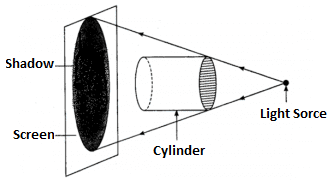If we throw the light on the cylinder from its circular side then we will get the image of a circle.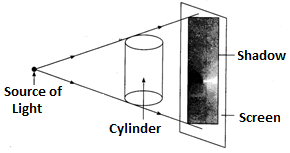If we throw the light on the cylinder while it is on standing position then its shadow will be rectangular in shape.

3. Viewing from different angles to get different views

All the 3D objects have a different view if we see them from different sides. By seeing them from different angles we can observe them easily.In the above figure, we will have a different top view, front view and side view but we get the information about the shape of the figure by observing them.
```### Course Features

• 728 Video Lectures
• Revision Notes
• Previous Year Papers
• Mind Map
• Study Planner
• NCERT Solutions
• Discussion Forum
• Test paper with Video Solution